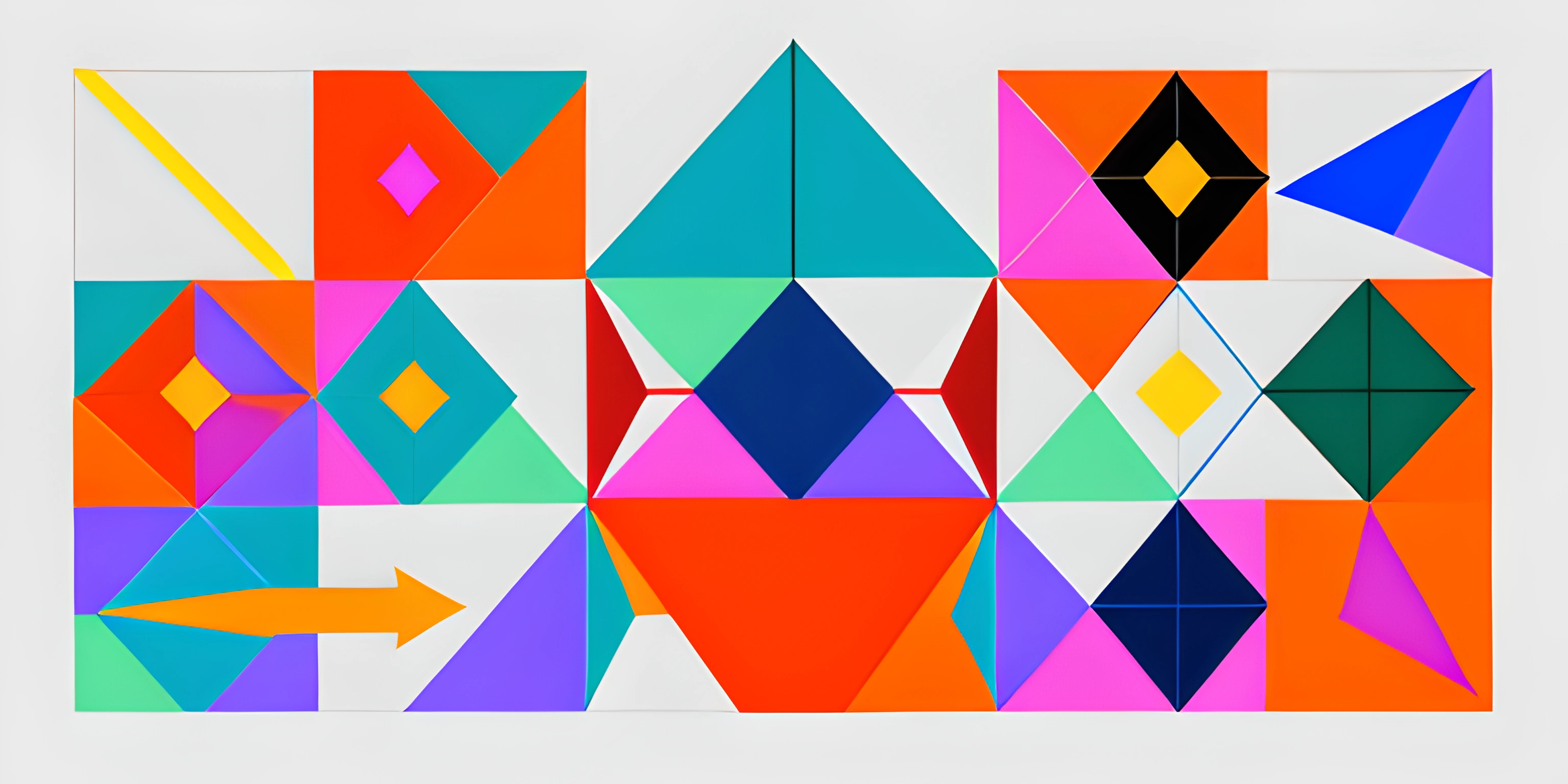# P5.js Vector ClassNote: this page has been created with the use of AI. Please take caution, and note that the content of this page does not necessarily reflect the opinion of Cratecode.

P5.js is a powerful library for creating graphics and interactive animations in the browser using JavaScript. One of the core features of p5.js is its ability to work with vectors. Vectors are essential when dealing with motion, physics, or anything involving position and direction. The p5.js Vector class provides a convenient way to handle vectors in both 2D and 3D space.

## What is a Vector?

A vector is a mathematical object that has both magnitude (length) and direction. In computer graphics and p5.js, vectors are typically represented as points in 2D or 3D space, with x, y, and sometimes z coordinates. Vectors are often used for tasks such as moving objects, calculating distances, and handling physics simulations.

## Creating a Vector in p5.js

To create a vector in p5.js, you can use the `createVector()` function, which returns a new instance of the p5.Vector class.

``let myVector = createVector(x, y, z);``

The `x`, `y`, and `z` arguments represent the vector's components. The `z` argument is optional, and when not provided, a 2D vector is created. Here's an example:

``let position = createVector(100, 200);``

This creates a 2D vector with x-coordinate 100 and y-coordinate 200.

## Vector Operations

The p5.Vector class provides various methods for performing common vector operations. Some of these include:

You can add or subtract two vectors using the `add()` and `sub()` methods. These methods modify the original vector and return it.

``````let v1 = createVector(10, 20);
let v2 = createVector(30, 40);

v1.add(v2); // v1 is now (40, 60)
v1.sub(v2); // v1 is now (10, 20)``````

### Scalar Multiplication and Division

You can multiply or divide a vector by a scalar (a single number) using the `mult()` and `div()` methods. These methods modify the original vector and return it.

``````let v = createVector(10, 20);

v.mult(2); // v is now (20, 40)
v.div(2); // v is now (10, 20)``````

### Magnitude

The magnitude (length) of a vector can be calculated using the `mag()` method.

``````let v = createVector(3, 4);
let magnitude = v.mag(); // magnitude is 5``````

### Normalization

To convert a vector to a unit vector (a vector with length 1), you can use the `normalize()` method.

``````let v = createVector(3, 4);
v.normalize(); // v is now approximately (0.6, 0.8)``````

## Using Vectors in p5.js Sketches

Vectors are commonly used in p5.js sketches to handle object positions, velocities, and accelerations. Here's an example of using vectors to move a circle across the canvas:

``````let position;
let velocity;

function setup() {
createCanvas(400, 400);
position = createVector(width / 2, height / 2);
velocity = createVector(2, 3);
}

function draw() {
background(0);

if (position.x > width || position.x < 0) {
velocity.x *= -1;
}

if (position.y > height || position.y < 0) {
velocity.y *= -1;
}

ellipse(position.x, position.y, 50);
}``````

In this sketch, we use the `position` vector to represent the circle's position and the `velocity` vector to represent its speed and direction. By adding the velocity to the position each frame, we create smooth motion. When the circle reaches the canvas edges, we reverse its velocity to make it bounce off.

Get creative and experiment with the p5.js Vector class to bring life to your sketches!